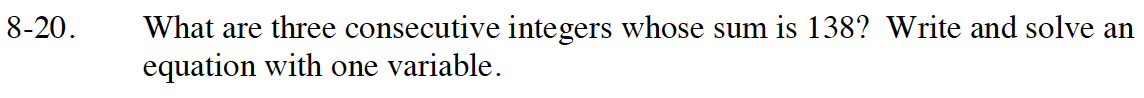### Home > CC4 > Chapter 8 > Lesson 8.1.1 > Problem8-20

8-20.

What are three consecutive integers whose sum is 138? Write and solve an equation with one variable. Homework Help ✎Let x = the first integer. How can you represent the second integer in terms of x? How about the third integer?

first integer + second integer + third integer = 138